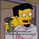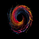‎267‎ مشاهدة
267
Just a mod to change the precision to zero (remove the useless digits e.g. indicator value 80.000000)
Also it appears that this indicator hasn't been published on the library yet.
```study(title = "Relative Momentum Index Elixium", shorttitle= "RMI Elixium", precision=0)
// Relative Momentum Index
//
// Roger Altman, February 1993, "Technical Analysis of Stocks & Commodities"
//
// Rewrite for Tradingview "Pine Script" by Kocurekc, April 2014
// Code is provided as public domain, no warranty

//******************************Using***************************
//Inputs - EMA averaging length
//Inputs - Momentum, lookback period for increase or decrease
//Option - Upper Bound Line, which sets the upper fill level
//Option - Lower Bound Line, which sets the lower fill level
//******************************END*****************************

Len = input(title="EMA Averaging Length", type=integer, defval=20, minval=1)
Mom = input(title="Lookback for Momentum", type=integer, defval=5, minval=1)
OVB = input(title="Top Boundary", type=integer, defval=80, minval=51, maxval=100)
OVS = input(title="Bottom Boundary", type=integer, defval=20, minval=0, maxval=49)
InA = input(title="SMA Trendline", type=bool, defval=false)
smaLen = input(title="SMA Period", type=integer, defval=10, minval=1)

emaInc = ema(max(close - close[Mom], 0), Len)
emaDec = ema(max(close[Mom] - close, 0), Len)
RMI = emaDec == 0 ? 0 : 100 - 100 / (1 + emaInc / emaDec)

p1 = plot(RMI >= OVB ? RMI : OVB, color=green)
p2 = plot(OVB, title='OverB', color=green)
p3 = plot(OVS, title='OverS', color=red)
p4 = plot(RMI <= OVS ? RMI : OVS, color=red)
hline(50, linestyle=dashed)

plot(RMI, color=black)
plot(InA?sma(RMI,smaLen):na)

fill(p1, p2, color=green, transp=50)
fill(p3, p4, color=red, transp=50)```

## التعليقاتIt was the wild west days of pine-scripting back then...I still like this indicator...must be a visual thing
ردThe RMI had been posted by Kocurekc 15 months ago:

And TheLark had posted it 17 months ago (but I think the version by Kocurekc, which you also posted looks much better):